Anzeige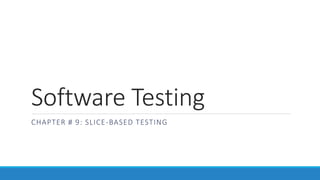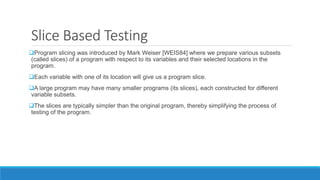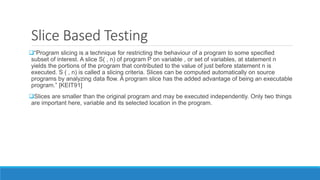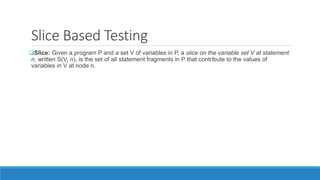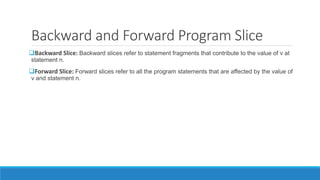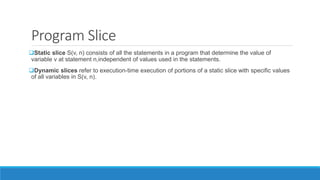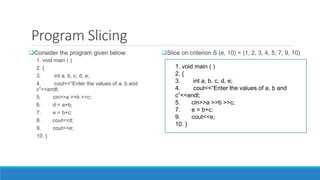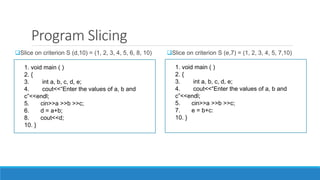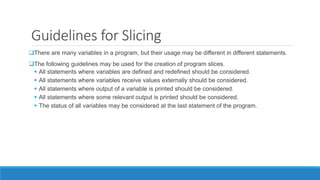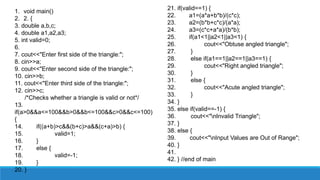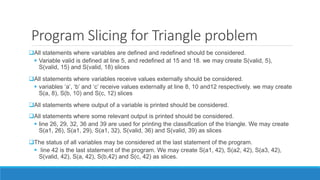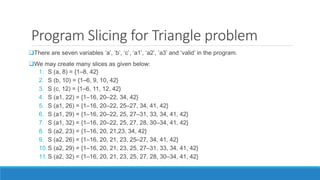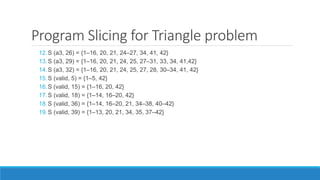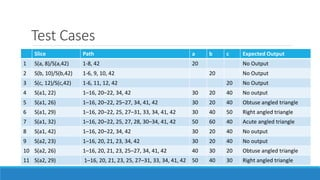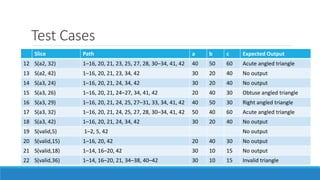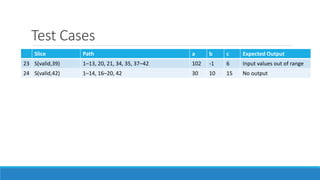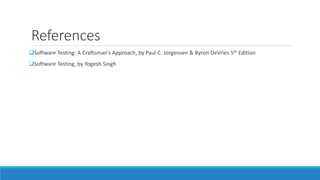1 von 18
Anzeige

### 1672734902268_Slice based testing.pptx

1. Software Testing CHAPTER # 9: SLICE-BASED TESTING
2. Slice Based Testing Program slicing was introduced by Mark Weiser [WEIS84] where we prepare various subsets (called slices) of a program with respect to its variables and their selected locations in the program. Each variable with one of its location will give us a program slice. A large program may have many smaller programs (its slices), each constructed for different variable subsets. The slices are typically simpler than the original program, thereby simplifying the process of testing of the program.
3. Slice Based Testing “Program slicing is a technique for restricting the behaviour of a program to some specified subset of interest. A slice S( , n) of program P on variable , or set of variables, at statement n yields the portions of the program that contributed to the value of just before statement n is executed. S ( , n) is called a slicing criteria. Slices can be computed automatically on source programs by analyzing data flow. A program slice has the added advantage of being an executable program.” [KEIT91] Slices are smaller than the original program and may be executed independently. Only two things are important here, variable and its selected location in the program.
4. Slice Based Testing Slice: Given a program P and a set V of variables in P, a slice on the variable set V at statement n, written S(V, n), is the set of all statement fragments in P that contribute to the values of variables in V at node n.
5. Backward and Forward Program Slice Backward Slice: Backward slices refer to statement fragments that contribute to the value of v at statement n. Forward Slice: Forward slices refer to all the program statements that are affected by the value of v and statement n.
6. Program Slice Static slice S(v, n) consists of all the statements in a program that determine the value of variable v at statement n,independent of values used in the statements. Dynamic slices refer to execution-time execution of portions of a static slice with specific values of all variables in S(v, n).
7. Static and Dynamic Slice 1. int z=10; 2. int n; 3. cin>>n; 4. int sum=0; 5. if (n>10) 6. sum = sum + n; 7. else 8. sum = sum – n; 9. cout <<sum; Static slice for variable sum 2,3,4,5,6,7,8,9 int n; cin>>n; int sum=0; if (n>10) sum = sum + n; else sum = sum – n; cout <<sum; Dynamic slice for variable sum when n= 15 2,3,4,5,6,9 int n; cin>>n; int sum=0; if (n>10) sum = sum + n; cout <<sum;
8. Program Slicing Consider the program given below: 1. void main ( ) 2. { 3. int a, b, c, d, e; 4. cout<<“Enter the values of a, b and c”<<endl; 5. cin>>a >>b >>c; 6. d = a+b; 7. e = b+c: 8. cout<<d; 9. cout<<e; 10. } Slice on criterion S (e, 10) = (1, 2, 3, 4, 5, 7, 9, 10) 1. void main ( ) 2. { 3. int a, b, c, d, e; 4. cout<<“Enter the values of a, b and c”<<endl; 5. cin>>a >>b >>c; 7. e = b+c: 9. cout<<e; 10. }
9. Program Slicing Slice on criterion S (e,7) = (1, 2, 3, 4, 5, 7,10) 1. void main ( ) 2. { 3. int a, b, c, d, e; 4. cout<<“Enter the values of a, b and c”<<endl; 5. cin>>a >>b >>c; 7. e = b+c: 10. } Slice on criterion S (d,10) = (1, 2, 3, 4, 5, 6, 8, 10) 1. void main ( ) 2. { 3. int a, b, c, d, e; 4. cout<<“Enter the values of a, b and c”<<endl; 5. cin>>a >>b >>c; 6. d = a+b; 8. cout<<d; 10. }
10. Guidelines for Slicing There are many variables in a program, but their usage may be different in different statements. The following guidelines may be used for the creation of program slices.  All statements where variables are defined and redefined should be considered.  All statements where variables receive values externally should be considered.  All statements where output of a variable is printed should be considered.  All statements where some relevant output is printed should be considered.  The status of all variables may be considered at the last statement of the program.
11. 1. void main() 2. 2. { 3. double a,b,c; 4. double a1,a2,a3; 5. int valid=0; 6. 7. cout<<"Enter first side of the triangle:"; 8. cin>>a; 9. cout<<"Enter second side of the triangle:"; 10. cin>>b; 11. cout<<"Enter third side of the triangle:"; 12. cin>>c; /*Checks whether a triangle is valid or not*/ 13. if(a>0&&a<=100&&b>0&&b<=100&&c>0&&c<=100) { 14. if((a+b)>c&&(b+c)>a&&(c+a)>b) { 15. valid=1; 16. } 17. else { 18. valid=-1; 19. } 20. } 21. if(valid==1) { 22. a1=(a*a+b*b)/(c*c); 23. a2=(b*b+c*c)/(a*a); 24. a3=(c*c+a*a)/(b*b); 25. if(a1<1||a2<1||a3<1) { 26. cout<<"Obtuse angled triangle"; 27. } 28. else if(a1==1||a2==1||a3==1) { 29. cout<<"Right angled triangle"; 30. } 31. else { 32. cout<<"Acute angled triangle"; 33. } 34. } 35. else if(valid==-1) { 36. cout<<"nInvalid Triangle"; 37. } 38. else { 39. cout<<"nInput Values are Out of Range"; 40. } 41. 42. } //end of main
12. Program Slicing for Triangle problem All statements where variables are defined and redefined should be considered.  Variable valid is defined at line 5, and redefined at 15 and 18. we may create S(valid, 5), S(valid, 15) and S(valid, 18) slices All statements where variables receive values externally should be considered.  variables ‘a’, ‘b’ and ‘c’ receive values externally at line 8, 10 and12 respectively. we may create S(a, 8), S(b, 10) and S(c, 12) slices All statements where output of a variable is printed should be considered. All statements where some relevant output is printed should be considered.  line 26, 29, 32, 36 and 39 are used for printing the classification of the triangle. We may create S(a1, 26), S(a1, 29), S(a1, 32), S(valid, 36) and S(valid, 39) as slices The status of all variables may be considered at the last statement of the program.  line 42 is the last statement of the program. We may create S(a1, 42), S(a2, 42), S(a3, 42), S(valid, 42), S(a, 42), S(b,42) and S(c, 42) as slices.
13. Program Slicing for Triangle problem There are seven variables ‘a’, ‘b’, ‘c’, ‘a1’, ‘a2’, ‘a3’ and ‘valid’ in the program. We may create many slices as given below: 1. S (a, 8) = {1–8, 42} 2. S (b, 10) = {1–6, 9, 10, 42} 3. S (c, 12) = {1–6, 11, 12, 42} 4. S (a1, 22) = {1–16, 20–22, 34, 42} 5. S (a1, 26) = {1–16, 20–22, 25–27, 34, 41, 42} 6. S (a1, 29) = {1–16, 20–22, 25, 27–31, 33, 34, 41, 42} 7. S (a1, 32) = {1–16, 20–22, 25, 27, 28, 30–34, 41, 42} 8. S (a2, 23) = {1–16, 20, 21,23, 34, 42} 9. S (a2, 26) = {1–16, 20, 21, 23, 25–27, 34, 41, 42} 10.S (a2, 29) = {1–16, 20, 21, 23, 25, 27–31, 33, 34, 41, 42} 11.S (a2, 32) = {1–16, 20, 21, 23, 25, 27, 28, 30–34, 41, 42}
14. Program Slicing for Triangle problem 12.S (a3, 26) = {1–16, 20, 21, 24–27, 34, 41, 42} 13.S (a3, 29) = {1–16, 20, 21, 24, 25, 27–31, 33, 34, 41,42} 14.S (a3, 32) = {1–16, 20, 21, 24, 25, 27, 28, 30–34, 41, 42} 15.S (valid, 5) = {1–5, 42} 16.S (valid, 15) = {1–16, 20, 42} 17.S (valid, 18) = {1–14, 16–20, 42} 18.S (valid, 36) = {1–14, 16–20, 21, 34–38, 40–42} 19.S (valid, 39) = {1–13, 20, 21, 34, 35, 37–42}
15. Test Cases Slice Path a b c Expected Output 1 S(a, 8)/S(a,42) 1-8, 42 20 No Output 2 S(b, 10)/S(b,42) 1-6, 9, 10, 42 20 No Output 3 S(c, 12)/S(c,42) 1-6, 11, 12, 42 20 No Output 4 S(a1, 22) 1–16, 20–22, 34, 42 30 20 40 No output 5 S(a1, 26) 1–16, 20–22, 25–27, 34, 41, 42 30 20 40 Obtuse angled triangle 6 S(a1, 29) 1–16, 20–22, 25, 27–31, 33, 34, 41, 42 30 40 50 Right angled triangle 7 S(a1, 32) 1–16, 20–22, 25, 27, 28, 30–34, 41, 42 50 60 40 Acute angled triangle 8 S(a1, 42) 1–16, 20–22, 34, 42 30 20 40 No output 9 S(a2, 23) 1–16, 20, 21, 23, 34, 42 30 20 40 No output 10 S(a2, 26) 1–16, 20, 21, 23, 25–27, 34, 41, 42 40 30 20 Obtuse angled triangle 11 S(a2, 29) 1–16, 20, 21, 23, 25, 27–31, 33, 34, 41, 42 50 40 30 Right angled triangle
16. Test Cases Slice Path a b c Expected Output 12 S(a2, 32) 1–16, 20, 21, 23, 25, 27, 28, 30–34, 41, 42 40 50 60 Acute angled triangle 13 S(a2, 42) 1–16, 20, 21, 23, 34, 42 30 20 40 No output 14 S(a3, 24) 1–16, 20, 21, 24, 34, 42 30 20 40 No output 15 S(a3, 26) 1–16, 20, 21, 24–27, 34, 41, 42 20 40 30 Obtuse angled triangle 16 S(a3, 29) 1–16, 20, 21, 24, 25, 27–31, 33, 34, 41, 42 40 50 30 Right angled triangle 17 S(a3, 32) 1–16, 20, 21, 24, 25, 27, 28, 30–34, 41, 42 50 40 60 Acute angled triangle 18 S(a3, 42) 1–16, 20, 21, 24, 34, 42 30 20 40 No output 19 S(valid,5) 1–2, 5, 42 No output 20 S(valid,15) 1–16, 20, 42 20 40 30 No output 21 S(valid,18) 1–14, 16–20, 42 30 10 15 No output 22 S(valid,36) 1–14, 16–20, 21, 34–38, 40–42 30 10 15 Invalid triangle
17. Test Cases Slice Path a b c Expected Output 23 S(valid,39) 1–13, 20, 21, 34, 35, 37–42 102 -1 6 Input values out of range 24 S(valid,42) 1–14, 16–20, 42 30 10 15 No output
18. References Software Testing: A Craftsman’s Approach, by Paul C. Jorgensen & Byron DeVries 5th Edition Software Testing, by Yogesh Singh
Anzeige